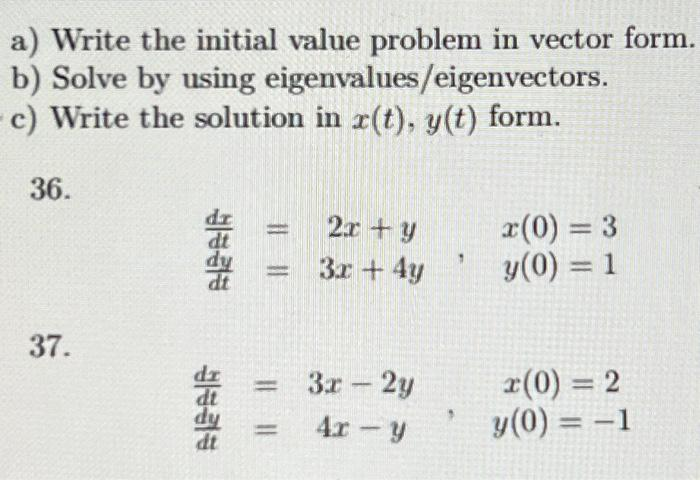# (Solved): 37) a) Write the initial value problem in vector form. b) Solve by using eigenvalues/eigenvectors. c ...

37)a) Write the initial value problem in vector form. b) Solve by using eigenvalues/eigenvectors. c) Write the solution in form. 36. 37.

We have an Answer from Expert

a) To write the initial value problems in vector form, we introduce a vector function:

For problem 36, the system of differential equations can be written in vector form as:

with the initial condition:

Similarly, for problem 37, the system of differential equations can be written in vector form as:

with the initial condition:

b) To solve these systems using eigenvalues and
eigenvectors, we first find the eigenvalues and
We have an Answer from Expert